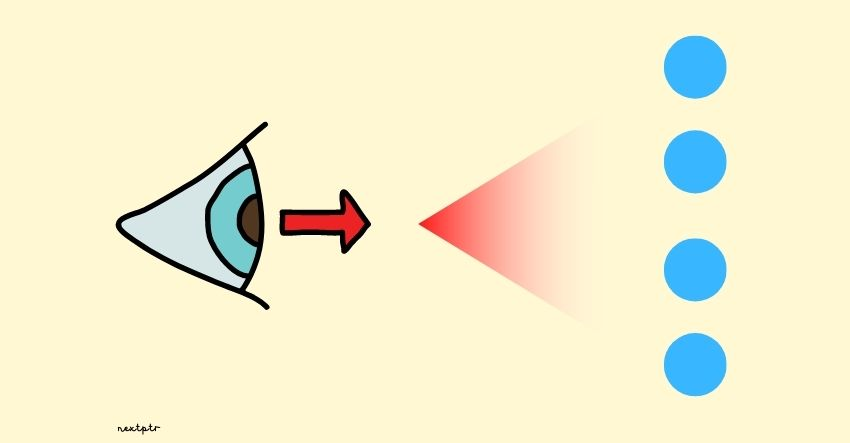# The std::span as a view of an array## Introduction

An instance of std::span<`T`> is a lightweight object that can refer to a contiguous sequence of objects starting at index zero. std::span is added to the standard library in C++20. However, it has been available to C++ developers as part of the GSL for quite some time now.

Conceptually, a span is a non-owning view of an underlying sequence or array. Essentially, a span has two data members: a pointer and size. But, span is not just a plain struct. It also serves as an abstract sequential container by providing several standard container methods (e.g., begin, end, front, back), which makes it quite useful to work with the standard library.

Let's take an example. The function add() takes an std::span<`int`> and iterates over it to calculate the sum of elements, as shown:

``````int64_t add(std::span<int> s) {
int64_t sum = 0;
for(auto i : s) //iterate over s
sum += i;
return sum;
}
``````

If it weren't for the span, the add() would have to take pointer and length parameters, and we would not be able to use the range-based for loop. Also, the function's code is bound-safe.

std::span provides several constructors. But for the most part, it can be constructed with an array or explicitly with a combination of pointer and size. The add() can be called with different sequences as follows:

``````/* An array. The size is implicit. */
int iArr[] = {1,1,1,1};
std::cout << add(iArr) << "\n"; //4

/* A dynamic array.
Requires explicit pointer and size to construct span. */
int* iPtr = new int;
iPtr = iPtr = iPtr = iPtr = 1;
std::cout << add({iPtr, 4}) << "\n"; //4

/* An std::array */
std::array<int, 4> isArr = {1,1,1,1};
std::cout << add(isArr) << "\n"; //4

/* A std::vector.
Requires explicit pointer and size to construct span.*/
std::vector<int> iVec = {1,1,1,1};
std::cout << add({&iVec, 4}) << "\n"; //4
``````

## The Question: A span on a section of an array

Consider a large `int` array, as shown below:

``````int largeArr;
``````

We want to invoke add() only on a portion of this array: 100 elements from largeArr to largeArr. In real-life problems, this could be done to invoke a function in parallel (in multiple threads) on smaller pieces of an array to speed up processing.

Select below all the correct ways to invoke the add() on the 100 elements of the array, starting at index 400 (check `Explanations` for details):

## Finally

std::span<`T`> is a read-write view on a sequence or array. However, you can always create a read-only span by using std::span<`const T`>. A span can be used as a view on an std::vector (or a part of std::vector) too. But we cannot use span on std::list or std::deque because they are not contiguous containers.